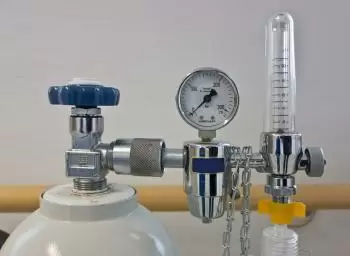# State and Explain Avogadro’s Law: Applications and FormulaAvogadro's Law is one of the experimental laws of gases, which in physics establishes a relationship between volume and the number of atoms or molecules that make up a gas.

The name of the law was established after Amadeo Avogadro, who, in 1812, raised his hypothesis. Avogadro hypothesized that two given samples of an ideal gas of equal volumes and under the same temperature and pressure conditions contain the same number of particles.

In reality, the behavior of real gases is slightly different from that of ideal gases. Although Avogadro's law only approximately holds, it is still a helpful approximation for chemists.

Avogadro's principle explains Gay-Lussac's law and Dalton's atomic model.

In 1983, Émile Clapeyron formulated the ideal gas law by combining Boyle's, Charles's, and Gay-Lussac's laws and Avogadro's.

Avogadro's law is one of the fundamental gas laws. The law establishes that: “all the cases with the same conditions of pressure, volume, and temperature, have the same number of moles.”

For example, let's take identical volumes of nitrogen and hydrogen in the gaseous state with the same pressure and temperature conditions. We observe that they contain the same number of molecules behaving according to the ideal gas laws.

This law states that all gases under the same temperature and pressure conditions have the same amount of substance proportional to their occupied volume.

Mathematically, the formula for Avogadro's law can be expressed as follows:Where:

• V1 and V2 are the volumes of two different gases

• n1 and n2 are the amounts of gas substance (measured in moles) of both gases.

As can be seen, the volumes are directly proportional to the moles of gas of each substance.

The volume occupied is called standard molar volume if the gas is at standard temperature and pressure.

Amedeo Avogadro, in his article of 1811, raised several hypotheses:

• First, the same volume of gases contains the same number of molecules under the same temperature and pressure conditions.

• Gaseous elements, such as hydrogen, nitrogen, and oxygen, are made up of diatomic molecules.

• The molecules are tiny compared to the space that separates them in gases.

According to Avogadro, in a chemical reaction, a reactant molecule must react with one or more molecules of another reactant, giving rise to one or more molecules of the product. In contrast, a molecule cannot react with a nonwhole number of molecules, already that the minimum unit of a reagent is the molecule. Therefore, a ratio of simple integers must exist between the molecules of the reactants and between these molecules and those of the product.

For this reason, there must be a direct relationship between these volumes of gases and the number of molecules they contain. To explain this law, Avogadro proposed the hypothesis that the molecules of most of the most common elementary gases ( hydrogen, nitrogen, oxygen, chlorine, etc.) were diatomic. Therefore, through chemical reactions, they can be separated into two atoms.

Since the molecules of different elements have different volumes, it is necessary to assume that they do not occupy the entire volume of the gas touching each other.

Avogadro's number (or Avogadro's constant) is a physical constant representing the number of atoms or molecules in one mole of a substance. It is expressed with the capital letter N and approximates 6.02 x 1023.

For example, a mole of carbon has 6.022 x 1023 atoms, and its mass is approximately 12 grams (the carbon molar mass is 12.01070 ± 0.00080 g/mol).

## Application of Avogadro's Law: Why Is It Important?

The law is essential because it allows you to calculate the amount of a substance from the number of moles of gas or its mass. It can also be used to convert between different concentration units, such as molality, molarity, and the atomicity of gases.

Also, Avogadro's Law can calculate the amount of gas in a container from the number of moles or the pressure. It can also determine the size of particles suspended in a liquid.